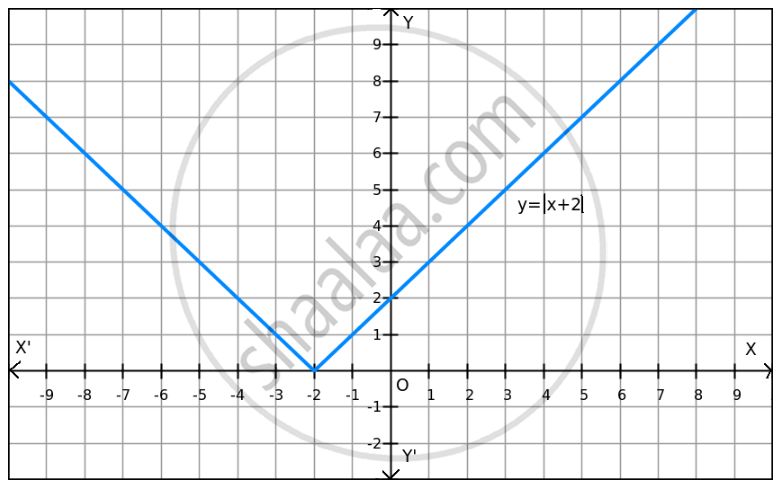PUC Karnataka Science Class 12Department of Pre-University Education, Karnataka
Share

F(X)=| X+2 | on R. - PUC Karnataka Science Class 12 - Mathematics

Question

f(x)=| x+2 | on R .

Solution

Given: f(x) = $\left| x + 2 \right|$

Now,

$\left| x + 2 \right| \geq 0$ for all x $\in$ R

Thus, f(x) $\geq$ 0 for all x $\in$ RTherefore, the minimum value of f at x = $-$ 2 is 0.

Since f(x) can be enlarged, the maximum value does not exist, which is evident in the graph also.

Hence, the function f does not have a maximum value.

Is there an error in this question or solution?

Video TutorialsVIEW ALL 

Solution F(X)=| X+2 | on R. Concept: Graph of Maxima and Minima.
S